# How to Count Unique Dates in Excel

This post will guide you how to count unique dates in a given range in Excel 2013/2016 or Excel office 365. How do I count the unique dates in a list of dates with some duplicate dates using a formula in Excel. And you can do this by a formula based on the SUMPRODUCT function and the COUNTIF function in an older version of Excel 2013/2016. You can also do it using UNIQUE function in the latest Excel 365.

Assuming you have a list of data range B1:B6 in your worksheet and you wish to count the number of unique dates using a formula. And the below introduction will show you the ways to achieve the result.

## Count Unique Dates in Old Version of Excel

If you are using older version of Excel, for example, Excel 2013 or Excel 2016, and you can use the COUNTIF function in combination with the SUMPRODUCT function to count unique dates with a formula like this:

`=SUMPRODUCT(1/COUNTIF(B1:B6,B1:B6))`

Let’s See How This Formula Works:

`=COUNTIF(B1:B6,B1:B6)`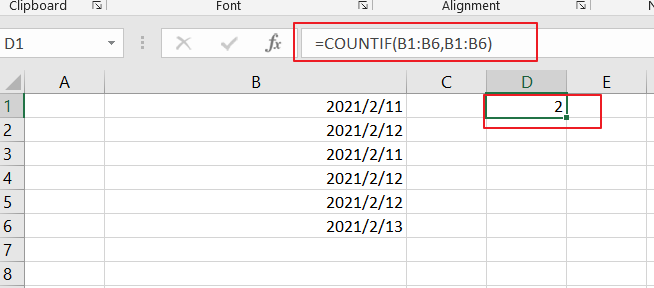The COUNTIF function will compare each date in the same data range and returns an array results that contain a count for every data in the given range. The result array is like this:

`{2;3;2;3;3;1}`
`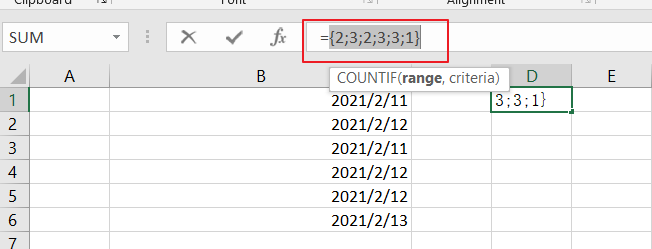`

Then you need to divide this array result by number 1 to return an array of fractional values like this:

`=1/COUNTIF(B1:B6,B1:B6)`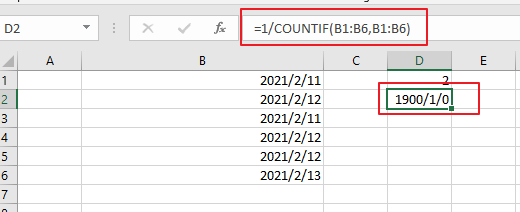`={0.5;0.333333333333333;0.5;0.333333333333333;0.333333333333333;1}`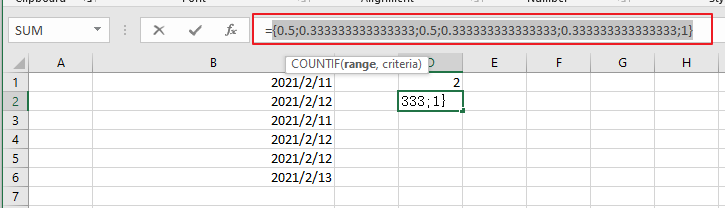Finally, you still need to add up the items in the above array. And you can use the SUMPRODUCT function to sum up the items in that array list. And it will return the total unique dates as 3.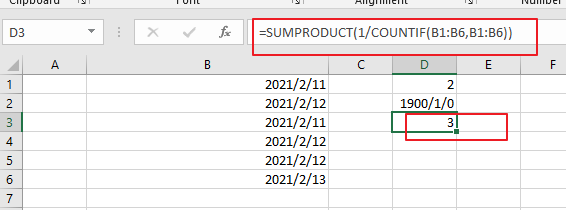You can also use another array formula based on the SUM Function and the FREQUENCY function to achieve the result of counting unique dates in the given date range. Like below:

`=SUM(--(FREQUENCY(B1:B6,B1:B6)>0))`

Then you need to put this formula into a blank cell and press “Ctrl +Shift +Enter” to make it as array formula.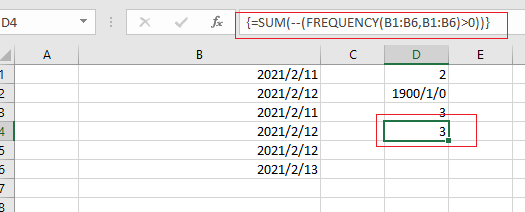Count Unique Dates in Excel 365

If you are working in Excel 365 and you can use the UNIQUE function in combination with the COUNT function to count unique dates in the given range cells in Excel.

The generic formula is like this:

`=COUNT(UNIQUE(B1:B6)`

The UNIQUE function can be used to extract a list of unique dates from a given range B1:B6, and then using the COUNT function to count numeric values. And if you wish to count both numeric and text values, you can replace the COUNT function with COUNTA function.

### Related Functions

• Excel SUMPRODUCT function
The Excel SUMPRODUCT function multiplies corresponding components in the given one or more arrays or ranges, and returns the sum of those products.The syntax of the SUMPRODUCT function is as below:= SUMPRODUCT (array1,[array2],…)…
• Excel COUNTIF function
The Excel COUNTIF function will count the number of cells in a range that meet a given criteria. This function can be used to count the different kinds of cells with number, date, text values, blank, non-blanks, or containing specific characters.etc.= COUNTIF (range, criteria)…
• Excel SUM function
The Excel SUM function will adds all numbers in a range of cells and returns the sum of these values. You can add individual values, cell references or ranges in excel.The syntax of the SUM function is as below:= SUM(number1,[number2],…)…
• Excel COUNT function
The Excel COUNT function counts the number of cells that contain numbers, and counts numbers within the list of arguments. It returns a numeric value that indicate the number of cells that contain numbers in a range…
• Excel FREQUENCY function
TThe Excel FREQUENCY function calculates how often values occur within a range of values. And it will return a vertical array of numbers.The syntax of the FREQUENCY function is as below:= FREQUENCY (data_array, bins_array)…
• Excel COUNTA function
The Excel COUNTA function counts the number of cells that are not empty in a range. The syntax of the COUNTA function is as below:= COUNTA(value1, [value2],…)…
• Excel UNIQUE function
The guide demonstrates how to use the UNIQUE function and dynamic arrays in Excel to create unique values.The syntax:=UNIQUE(array, [by col], [once exactly]) …